### 3.3.2.2 Flux Equations

The current density is discretized by a scheme which is frequently referred to as SCHARFETTER-GUMMEL discretization . The extension of the discretization to the flux equations stemming from the higher order moments of BOLTZMANN's equation is not beyond controversy, so different approaches can be found in the literature. In  it is assumed that the electron concentration is a known function of exponential shape. This strategy is refined in  where the variation of the electron concentration obtained from the discretization of the current density equation is used for discretizing the energy flux density. This text will follow the approach presented in , which is an extension of  and , where a generalized expression for the fluxes is used and no assumption about the variation of the carrier concentration is made.

By rewriting the flux equations (2.188) to (2.190)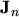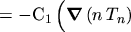(3.34)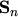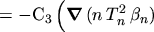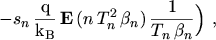(3.35)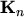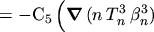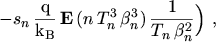(3.36)

a common functional form can be recognized. Therefore a general flux equation is introduced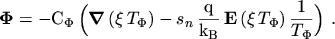(3.37)

The meanings of the generalized density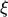and temperature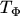is found by inspection: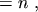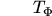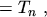(3.38)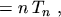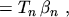(3.39)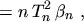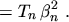(3.40)

By projecting eqn. (3.37) onto a grid line a one-dimensional differential equation is obtained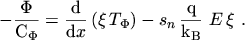(3.41)

To solve this equation the following assumptions have been made:

• constant general flux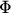,
• constant electric field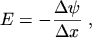(3.42)

• linear variation of the general temperature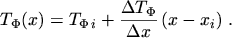(3.43)

The solution of eqn. (3.41) is found by multiplication with an integrating factor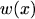and by sub-sequentially comparing the coefficients of the resulting equation with the total derivative of the product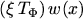: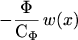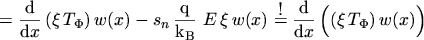(3.44)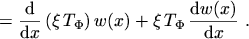(3.45)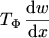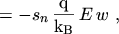(3.46)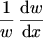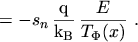(3.47)

This equation can be solved for the integrating factor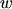, taking into account the assumptions (3.42) and (3.43):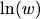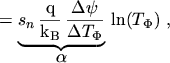(3.48)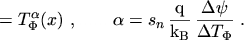(3.49)

Inserting the integrating factor into eqn. (3.44)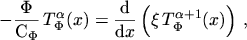(3.50)

and assuming that the fluxis constant between two grid points, eqn. (3.50) can be integrated from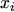to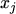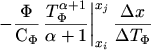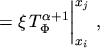(3.51)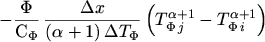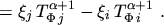(3.52)

Commonly eqn. (3.52) is rewritten using the BERNOULLI function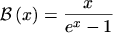(3.53)

Beginning with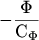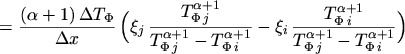(3.54)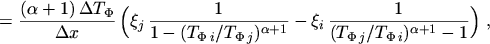(3.55)

and using the abbreviations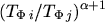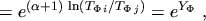(3.56)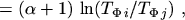(3.57)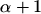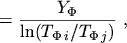(3.58)

the flux equation can be written as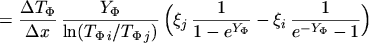(3.59)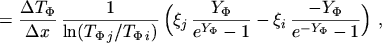(3.60)

or using the BERNOULLI function as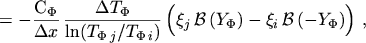(3.61)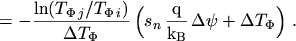(3.62)

The concept of assuming a constant flux density was first presented by SCHARFETTER and GUMMEL in the appendix of [51, p.73]. The assumption of a linear variation of the generalized temperatureby eqn. (3.43) can be interpreted as a straightforward extension of the SCHARFETTER-GUMMEL scheme.

An advantage of using BERNOULLI functions in the flux equations is that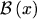is well defined at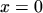.

Inserting the abbreviations (3.38) to (3.40) used forandyields the discretized flux equations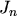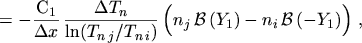(3.63)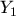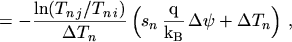(3.64)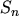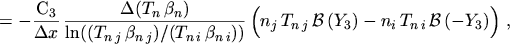(3.65)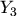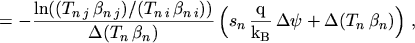(3.66)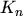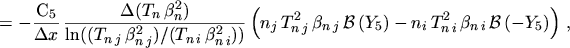(3.67)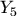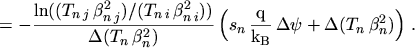(3.68)

M. Gritsch: Numerical Modeling of Silicon-on-Insulator MOSFETs PDF